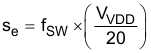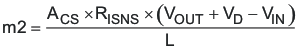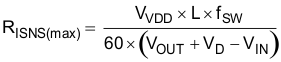SLVS861F August   2008  – June 2020

PRODUCTION DATA.

1. Features
2. Applications
3. Description
1.     Device Images
4. Revision History
5. Pin Configuration and Functions
6. Specifications
7. Detailed Description
1. 7.1 Overview
2. 7.2 Functional Block Diagram
3. 7.3 Feature Description
4. 7.4 Device Functional Modes
8. Application and Implementation
1. 8.1 Application Information
2. 8.2 Typical Application
1. 8.2.1 Design Requirements
2. 8.2.2 Detailed Design Procedure
3. 8.2.3 Application Curves
9. Power Supply Recommendations
10. 10Layout
11. 11Device and Documentation Support
1. 11.1 Device Support
2. 11.2 Documentation Support
3. 11.3 Related Links
5. 11.5 Electrostatic Discharge Caution
6. 11.6 Glossary
12. 12Mechanical, Packaging, and Orderable Information

• DGQ|10
• DGQ|10

#### 7.3.3 Current Sense and Subharmonic Instability

A characteristic of peak current-mode control results in a condition where the current control loop can exhibit instability. This results in alternating long and short pulses from the pulse-width modulator. The voltage loop maintains regulation and does not oscillate, but the output ripple voltage increases. The condition occurs only when the converter is operating in continuous conduction mode, and the duty cycle is 50% or greater. The cause of this condition is described in the Modeling, Analysis and Compensation of the Current-Mode Converter Application Report. The remedy for this condition is to apply a compensating ramp from the oscillator to the signal going to the pulse-width modulator. In the TPS40210-Q1 and TPS40211-Q1 devices, the oscillator ramp is applied in a fixed amount to the pulse-width modulator. The slope of the ramp is given in Equation 7.

Equation 7.To ensure that the converter does not enter into sub-harmonic instability, the slope of the compensating ramp signal must be at least half of the down slope of the current ramp signal. Because the compensating ramp is fixed in the TPS40210-Q1 and TPS40211-Q1 devices, this places a constraint on the selection of the current sense resistor.

The down slope of the current sense wave form at the pulse-width modulator is described in Equation 8.

Equation 8.Because the slope compensation ramp must be at least half, and preferably equal to, the down slope of the current sense waveform seen at the pulse-width modulator, a maximum value is placed on the current sense resistor when operating in continuous mode at 50% duty cycle or greater. For design purposes, some margin should be applied to the actual value of the current sense resistor. As a starting point, the actual resistor chosen should be 80% or less that the value calculated in Equation 9. This equation calculates the resistor value that makes the slope compensation ramp equal to one half of the current ramp downslope. Values no more than 80% of this result are acceptable.

Equation 9.where

• Se is the slope of the voltage compensating ramp applied to the pulse-width modulator in V/s
• fSW is the switching frequency in Hz
• VDD is the voltage at the VDD pin in V
• m2 is the down slope of the current sense waveform seen at the pulse-width modulator in V/s
• RISNS is the value of the current sense resistor in Ω
• VOUT is the converter output voltage VIN is the converter power stage input voltage
• VD is the drop across the diode in Figure 21

It is possible to increase the voltage compensation ramp slope by connecting the VDD pin to the output voltage of the converter instead of the input voltage as shown in Figure 21. This can help in situations where the converter design calls for a large ripple current value in relation to the desired output current limit setting.

NOTE

Connecting the VDD pin to the output voltage of the converter affects the start-up voltage of the converter since the controller undervoltage lockout (UVLO) circuit monitors the VDD pin and senses the input voltage less the diode drop before start-up. The effect is to increase the start-up voltage by the value of the diode voltage drop.

If an acceptable RISNS value is not available, the next higher value can be used and the signal from the resistor divided down to an acceptable level by placing another resistor in parallel with CISNS.